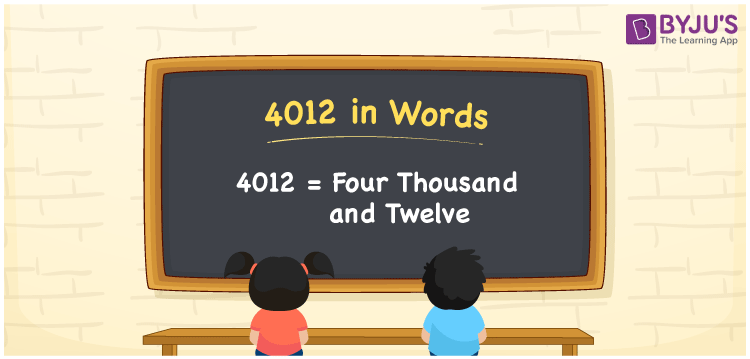# 4012 in Words

We can express 4012 in words as Four Thousand and Twelve. For example, Sushmita purchased a juicer mixer grinder worth Rs. 4012, then you can say, “Sushmita purchased a juicer mixer grinder worth Rupees Four Thousand and Twelve”. We can easily write the numerical name for 4012 using a place value chart. 4012 is a cardinal number since it shows the exact value of something. In this article, let us learn the method of converting the number 4012 into words.

 4012 in Words Four Thousand and Twelve Four Thousand and Twelve in numerical form 4012

## 4012 in English Words

Generally, we write the numbers in words using the letters of the English alphabet. Therefore, the number 4012 in English words is written as Four Thousand and Twelve.## How to Write 4012 in Words?

Let us make a chart of four columns since 4012 is a four-digit number. This is shown in the below table.

 Thousands Hundreds Tens Ones 4 0 1 2

Hence, we can write the expanded form as:

4 x Thousands + 0 x Hundred + 1 x Ten + 2 x One

= 4 x 1000 + 0 x 100 + 1 x 10 + 2 x 1

= 4000 + 0 + 10 + 2

= 4000 + 10 + 2

= 4012

= Four Thousand and Twelve

Therefore, 4012 in words is written as Four Thousand and Twelve

Interesting way of writing 4012 in words

4 = Four

40 = Forty

401 = Four Hundred and One

4012 = Four Thousand and Twelve

Thus, the word form of the number 4012 is Four Thousand and Twelve

4012 is a natural number that precedes 4013 and succeeds 4011

• 4012 in words – Four Thousand and Twelve
• Is 4012 an odd number? – No
• Is 4012 an even number? – Yes
• Is 4012 a perfect square number? – No
• Is 4012 a perfect cube number? – No
• Is 4012 a prime number? – No
• Is 4012 a composite number? – Yes

## Frequently Asked Questions on 4012 in Words

Q1

### Write 4012 in words.

4012 in words is written as Four Thousand and Twelve.
Q2

### Simplify 3000 + 1012, and express in words.

Simplifying 3000 + 1012, we get 4012. Hence, 4012 in words is Four Thousand and Twelve.
Q3

### What is the number name of 4012?

The number name of 4012 is Four Thousand and Twelve.